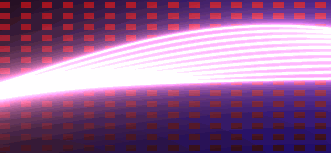```Shader "shadertoy/Waves" { //see https://www.shadertoy.com/view/4dsGzH
CGINCLUDE
#include "UnityCG.cginc"
#pragma target 3.0
struct vertOut {
float4 pos:SV_POSITION;
float4 srcPos;
};
vertOut vert(appdata_base v) {
vertOut o;
o.pos = mul (UNITY_MATRIX_MVP, v.vertex);
o.srcPos = ComputeScreenPos(o.pos);
return o;
}
fixed4 frag(vertOut i) : COLOR0 {
fixed3 COLOR1 = fixed3(0.0,0.0,0.3);
fixed3 COLOR2 = fixed3(0.5,0.0,0.0);
float BLOCK_WIDTH = 0.03;
float2 uv = (i.srcPos.xy/i.srcPos.w);
// To create the BG pattern
fixed3 final_color = fixed3(1.0);
fixed3 bg_color = fixed3(0.0);
fixed3 wave_color = fixed3(0.0);
float c1 = fmod(uv.x, 2.0* BLOCK_WIDTH);
c1 = step(BLOCK_WIDTH, c1);
float c2 = fmod(uv.y, 2.0* BLOCK_WIDTH);
c2 = step(BLOCK_WIDTH, c2);
bg_color = lerp(uv.x * COLOR1, uv.y * COLOR2, c1*c2);
// TO create the waves
float wave_width = 0.01;
uv = -1.0 + 2.0*uv;
uv.y += 0.1;
for(float i=0.0; i<10.0; i++) {
uv.y += (0.07 * sin(uv.x + i/7.0 + _Time.y));
wave_width = abs(1.0 / (150.0 * uv.y));
wave_color += fixed3(wave_width * 1.9, wave_width, wave_width * 1.5);
}
final_color = bg_color + wave_color;
return fixed4(final_color, 1.0);
}
ENDCG
Pass {
CGPROGRAM
#pragma vertex vert
#pragma fragment frag
#pragma fragmentoption ARB_precision_hint_fastest
ENDCG
}
}
FallBack Off
}
```

1. ComputeScreenPos的解析：

ComputeScreenPos在UnityCG.cginc文件中定义如下：

```// Projected screen position helpers
#define V2F_SCREEN_TYPE float4
inline float4 ComputeScreenPos (float4 pos) {
float4 o = pos * 0.5f;
#if defined(UNITY_HALF_TEXEL_OFFSET)
o.xy = float2(o.x, o.y*_ProjectionParams.x) + o.w * _ScreenParams.zw;
#else
o.xy = float2(o.x, o.y*_ProjectionParams.x) + o.w;
#endif
o.xy *= unity_NPOTScale.xy;
#endif
o.zw = pos.zw;
return o;
}
```

2. 背景的绘制

2.1) fmod用于求余数，比如fmod(1.5, 1.0) 返回0.5；

2.2) step用于大小的比较，step(a,x) :  0 if x<a; 1 if x>=a; 比如: step(1, 1.2), 返回1; step(1, 0.8) 返回0；

2.3) 结合fmod和step可以得到一个虚线的效果。 比如要得到虚线段长度为1的代码如下：

c1 = fmod(x, 2*width); c1=step(width,c1); //其中width为1

2.4)把2.3中的知识运用到2维，就可以计算出方块。

lerp函数的用法：lerp( a , b ,f )， f为百分数（取值范围［0，1］）；如果f为0，则lerp返回a，f为1，则返回b。f为0到1之间，就返回a到b之间的值。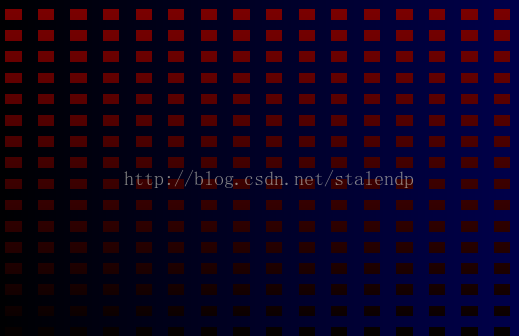3. 波纹的绘制

3.1 ) 坐标的转化

uv = -1.0 + 2.0*uv;  // 把原始的uv进行扩展和位移，得到新的uv。我们的操作就是在新的uv上进行的，最终显示时会映射到原来到uv，请参考下图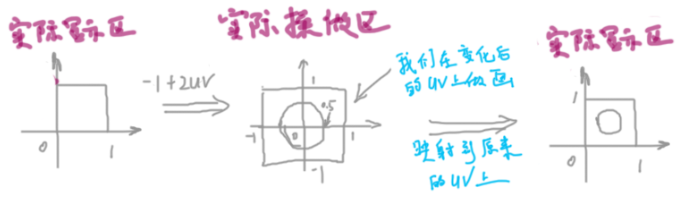3.2 )  画一条直线：

```wave_width = abs(1.0 / (50.0 * uv.y));
wave_color = fixed3(wave_width * 1.9, wave_width, wave_width * 1.5);
```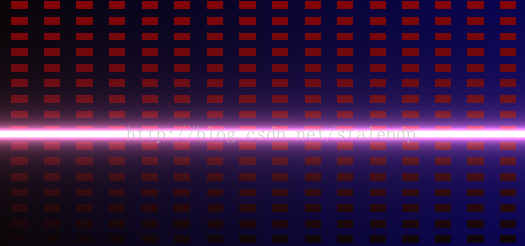3.3）把直线变为曲线，并使其动起来：

```uv.y += (0.07 * sin(uv.x*10 + _Time.y));
wave_width = abs(1.0 / (50.0 * uv.y));
wave_color = fixed3(wave_width * 1.9, wave_width, wave_width * 1.5);
```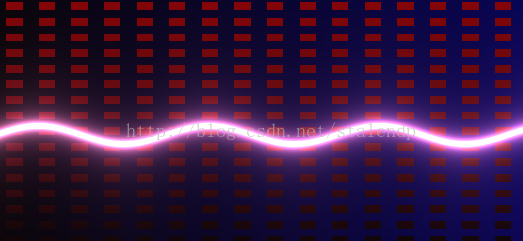3.4）多画几条曲线，形成波浪：

```for(float i=0.0; i<10.0; i++) {
uv.y += (0.07 * sin(uv.x + i/7.0 + _Time.y));
wave_width = abs(1.0 / (150.0 * uv.y));
wave_color += fixed3(wave_width * 1.9, wave_width, wave_width * 1.5);
}
```